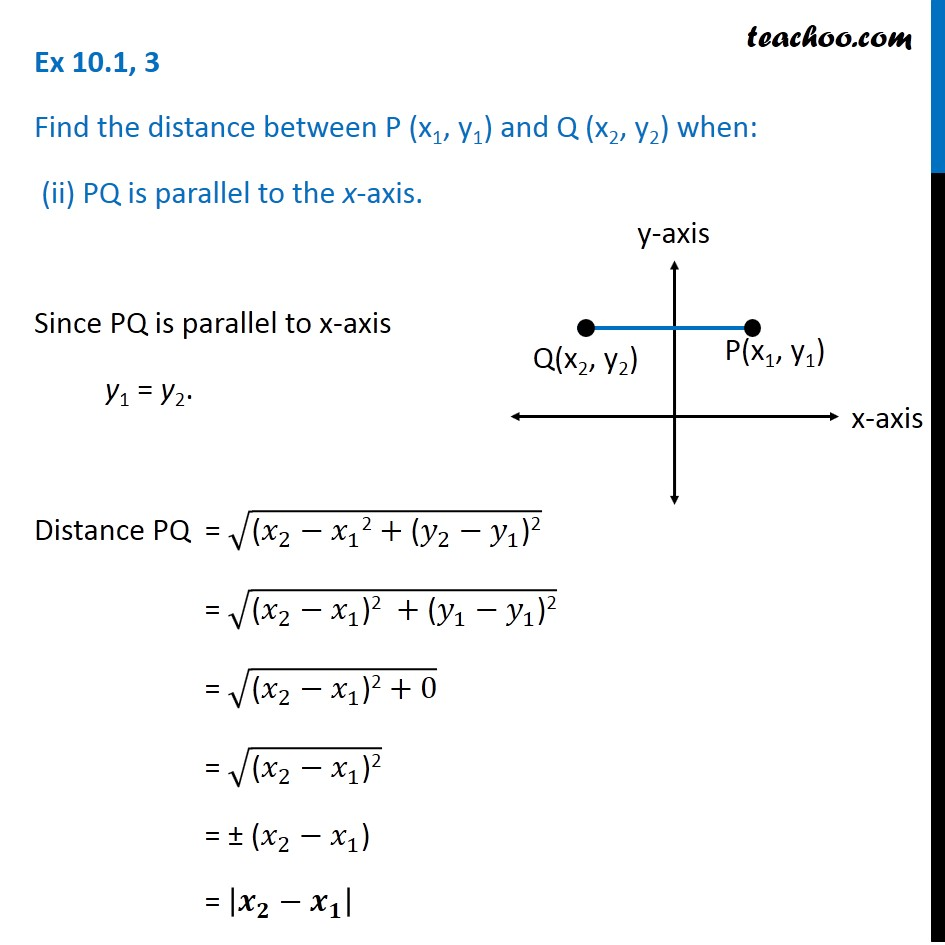Ex 9.1

Chapter 9 Class 11 Straight Lines
Serial order wiseLearn in your speed, with individual attention - Teachoo Maths 1-on-1 Class

### Transcript

Ex 9.1, 3 Find the distance between P (x1, y1) and Q (x2, y2) when: (ii) PQ is parallel to the x-axis. Since PQ is parallel to x-axis y1 = y2. Distance PQ = √("(" 𝑥_2−𝑥_1 "2" +"(" 𝑦_2−𝑦_1 ")2" ) = √("(" 𝑥_2−𝑥_1 ")2 " +"(" 𝑦_1−𝑦_1 ")2" ) = √("(" 𝑥_2−𝑥_1 ")2" +0) = √("(" 𝑥_2−𝑥_1 ")2" ) = ± (𝑥_2−𝑥_1) = |𝒙_𝟐−𝒙_𝟏 |